Next: Acknowledgments Up: Recursive Functions of Symbolic Previous: Another Formalism for Functions

# Flowcharts and Recursion

Since both the usual form of computer program and recursive function definitions are universal computationally, it is interesting to display the relation between them. The translation of recursive symbolic functions into computer programs was the subject of the rest of this report. In this section we show how to go the other way, at least in principle.

The state of the machine at any time during a computation is given by the values of a number of variables. Let these variables be combined into a vector
. Consider a program block with one entrance and one exit. It defines and is essentially defined by a certain function
that takes one machine configuration into another, that is,has the form
. Let us callthe associated function of the program block. Now let a number of such blocks be combined into a program by decision elements
that decide after each block is completed which block will be entered next. Nevertheless, let the whole program still have one entrance and one exit.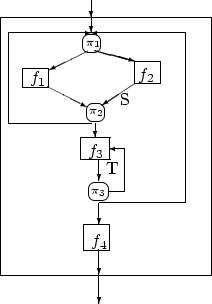Figure 5

We give as an example the flowcart of figure 5. Let us describe the function
that gives the transformation of the vectorbetween entrance and exit of the whole block. We shall define it in conjunction with the functions s(), and t[], which give the transformations thatundergoes between the points S and T, respectively, and the exit. We have
Given a flowchart with a single entrance and a single exit, it is easy to write down the recursive function that gives the transformation of the state vector from entrance to exit in terms of the corresponding functions for the computation blocks and the predicates of the branch. In general, we proceed as follows.

In figure 6, let
be an n-way branch point, and let
be the computations leading to branch points
. Let
be the function that transformsbetweenand the exit of the chart, and let
be the corresponding functions for
. We then write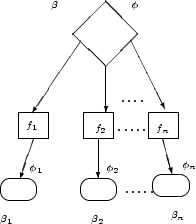Figure 6Next: Acknowledgments Up: Recursive Functions of Symbolic Previous: Another Formalism for Functions
John McCarthy
2006-08-13